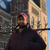# New to Qlik Sense

If you’re new to Qlik Sense, start with this Discussion Board and get up-to-speed quickly.

cancel
Showing results for
Did you mean:Creator

## Colormix no data normalized

Hi everyone,

I want to use the colormix2 function, but the field in question is not normalized. Is there a way to be able to normalize my field within the colormix function?

I would like to have a gradient from red to green.

For example: -1 = Red, and 1 Green

Thanks,

Livio

1 Solution

Accepted SolutionsChampion

Try this on the background color expression:

ColorMix1 ((1+Sign(2*(Sum({<[Scenario_DB] = {\$(=vPrimaryScen)}, [HFM.Year_DB] = {\$(=vPrimaryYear)}, [HFM.Month] = {\$(=vPrimaryMonth)}>}[HFM.Commercial Net Sales (DB)]/1000000)-RangeMin (top(total Sum({<[Scenario_DB] = {\$(=vPrimaryScen)}, [HFM.Year_DB] = {\$(=vPrimaryYear)}, [HFM.Month] = {\$(=vPrimaryMonth)}>}[HFM.Commercial Net Sales (DB)]/1000000),1,NoOfRows(total))))/(RangeMax (top(total Sum({<[Scenario_DB] = {\$(=vPrimaryScen)}, [HFM.Year_DB] = {\$(=vPrimaryYear)}, [HFM.Month] = {\$(=vPrimaryMonth)}>}[HFM.Commercial Net Sales (DB)]/1000000),1,NoOfRows(total)))-RangeMin (top(total Sum({<[Scenario_DB] = {\$(=vPrimaryScen)}, [HFM.Year_DB] = {\$(=vPrimaryYear)}, [HFM.Month] = {\$(=vPrimaryMonth)}>}[HFM.Commercial Net Sales (DB)]/1000000),1,NoOfRows(total))))-1)*Sqrt(Fabs((2*(Sum({<[Scenario_DB] = {\$(=vPrimaryScen)}, [HFM.Year_DB] = {\$(=vPrimaryYear)}, [HFM.Month] = {\$(=vPrimaryMonth)}>}[HFM.Commercial Net Sales (DB)]/1000000)-RangeMin (top(total Sum({<[Scenario_DB] = {\$(=vPrimaryScen)}, [HFM.Year_DB] = {\$(=vPrimaryYear)}, [HFM.Month] = {\$(=vPrimaryMonth)}>}[HFM.Commercial Net Sales (DB)]/1000000),1,NoOfRows(total))))/(RangeMax (top(total Sum({<[Scenario_DB] = {\$(=vPrimaryScen)}, [HFM.Year_DB] = {\$(=vPrimaryYear)}, [HFM.Month] = {\$(=vPrimaryMonth)}>}[HFM.Commercial Net Sales (DB)]/1000000),1,NoOfRows(total)))-RangeMin (top(total Sum({<[Scenario_DB] = {\$(=vPrimaryScen)}, [HFM.Year_DB] = {\$(=vPrimaryYear)}, [HFM.Month] = {\$(=vPrimaryMonth)}>}[HFM.Commercial Net Sales (DB)]/1000000),1,NoOfRows(total))))-1))))/2, ARGB(255, 0, 128, 0), ARGB(255, 255, 0, 0))

7 RepliesChampion

if you don't master the syntax of colormix() function is would suggest you to try the colormix_wizard that you can find on Qlikview desktopit will generate for you the code you need, just copy paste to qliksense.

It will save you a lot of timeCreator
Author

I'm using Qlik SenseChampion

Yes I Know, it is just a suggestion.

it is worth installing qlikview desktop to generate the colormix expression if you don't know how to write itCreator
Author

Are you able to generate for me the colormix function?

my value is:

Sum({<[Scenario_DB] = {\$(=vPrimaryScen)}, [HFM.Year_DB] = {\$(=vPrimaryYear)}, [HFM.Month] = {\$(=vPrimaryMonth)}>}[HFM.Commercial Net Sales (DB)]/1000000)  AS x

and i want a gradient color from Red to Green.

Thanks,

LivioChampion

Try this on the background color expression:

ColorMix1 ((1+Sign(2*(Sum({<[Scenario_DB] = {\$(=vPrimaryScen)}, [HFM.Year_DB] = {\$(=vPrimaryYear)}, [HFM.Month] = {\$(=vPrimaryMonth)}>}[HFM.Commercial Net Sales (DB)]/1000000)-RangeMin (top(total Sum({<[Scenario_DB] = {\$(=vPrimaryScen)}, [HFM.Year_DB] = {\$(=vPrimaryYear)}, [HFM.Month] = {\$(=vPrimaryMonth)}>}[HFM.Commercial Net Sales (DB)]/1000000),1,NoOfRows(total))))/(RangeMax (top(total Sum({<[Scenario_DB] = {\$(=vPrimaryScen)}, [HFM.Year_DB] = {\$(=vPrimaryYear)}, [HFM.Month] = {\$(=vPrimaryMonth)}>}[HFM.Commercial Net Sales (DB)]/1000000),1,NoOfRows(total)))-RangeMin (top(total Sum({<[Scenario_DB] = {\$(=vPrimaryScen)}, [HFM.Year_DB] = {\$(=vPrimaryYear)}, [HFM.Month] = {\$(=vPrimaryMonth)}>}[HFM.Commercial Net Sales (DB)]/1000000),1,NoOfRows(total))))-1)*Sqrt(Fabs((2*(Sum({<[Scenario_DB] = {\$(=vPrimaryScen)}, [HFM.Year_DB] = {\$(=vPrimaryYear)}, [HFM.Month] = {\$(=vPrimaryMonth)}>}[HFM.Commercial Net Sales (DB)]/1000000)-RangeMin (top(total Sum({<[Scenario_DB] = {\$(=vPrimaryScen)}, [HFM.Year_DB] = {\$(=vPrimaryYear)}, [HFM.Month] = {\$(=vPrimaryMonth)}>}[HFM.Commercial Net Sales (DB)]/1000000),1,NoOfRows(total))))/(RangeMax (top(total Sum({<[Scenario_DB] = {\$(=vPrimaryScen)}, [HFM.Year_DB] = {\$(=vPrimaryYear)}, [HFM.Month] = {\$(=vPrimaryMonth)}>}[HFM.Commercial Net Sales (DB)]/1000000),1,NoOfRows(total)))-RangeMin (top(total Sum({<[Scenario_DB] = {\$(=vPrimaryScen)}, [HFM.Year_DB] = {\$(=vPrimaryYear)}, [HFM.Month] = {\$(=vPrimaryMonth)}>}[HFM.Commercial Net Sales (DB)]/1000000),1,NoOfRows(total))))-1))))/2, ARGB(255, 0, 128, 0), ARGB(255, 255, 0, 0))Creator
Author

ThanksChampion

You're welcomeGood luckTags
Community Browser